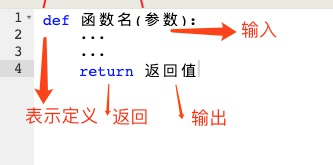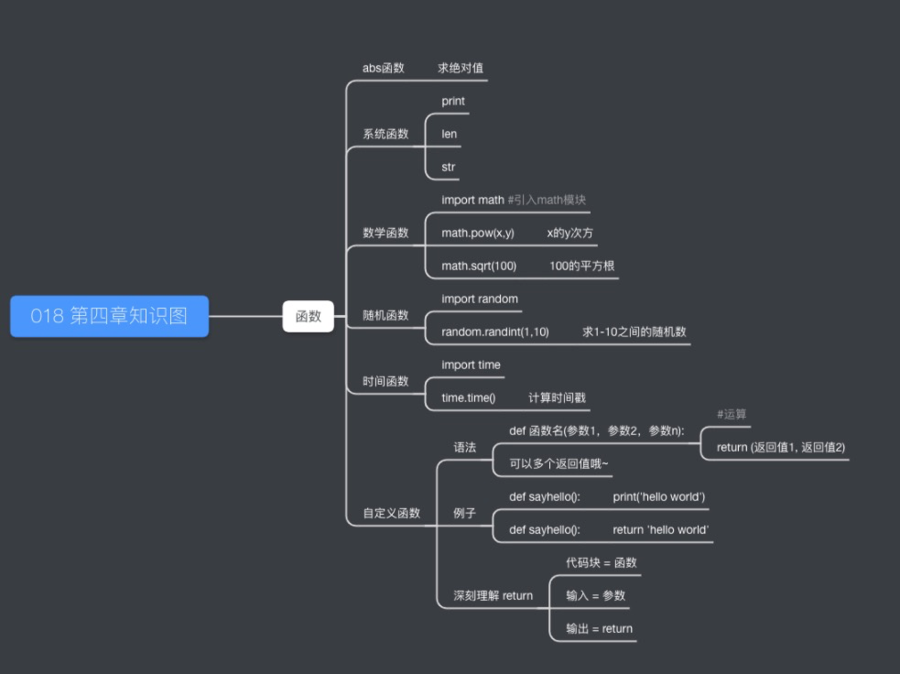《金哥百变Python零基础教程4》~函数与模块

# 本章知识点 :> `函数与模块`

p0总结在线看
p1总结在线看• 预告：我们将用 `函数` 来优化地铁问题！

`a = -1`
`jueduizhi = a`
`if a < 0:`
`jueduizhi = -a`

`a = -1`
`jueduizhi = abs(a)`

`abs(变量)` 就是求变量绝对值的函数，上面两种写法的效果是一样的。(思考：abs() 函数实现的语句是如何的？思路跟我们自己写应该一样吧。）

# print

• `print` `len` `str` 这几个运算（函数) 还记得吗?

• 除了 `+` `-` `*` `/` 这些符号运算之外的，用 `print` 这样的一个字母命名的 `运算` 都是 `函数`
• `print` 是这个函数的名字 （函数名)
• `print(s)` 我们用 `函数名(变量)` (两个括号包含要运算的变量) 的语法来 `调用` 函数，`调用` 意思其实就是运算
• `abs(a)` 语法也一样

• 易错点：函数的`参数`(参与运算的变量) 并非只能是一个
• 比如 ： `print("a", "bc", 123)`
• 这样的写法是可以的哦，具体函数要多少个`参数`（参与运算的变量）呢，每一个函数不一样（正如每个运算不一样）
试一下 在线Python

# 数学函数

• 函数的能力非常强大。
• 一组相关函数往往会放到一起，组成一个 `模块`, 比如数学相关函数，都放到 `math` 模块下
• `math` 有哪些函数呢？你能想到的数学相关函数都有！
• 要用数据函数，需要先`import math` 引入模块

• `import math`
`#然后才能调用数学函数`
• 随机相关的函数 `import random`

• 系统相关的函数(文件等等） `import os`

• 网络请求相关函数 `import requests`

• 机器学习相关函数 `import sklearn`

• 我之所以要在这写下一堆的 `import` 但一个具体函数也不写，其实就是为了表达， `函数` 是非常强大的！

• 挑几个数学函数看一看
• 1 x的y次方，即x**y
• `import math`
`math.pow(3,4)`
• 2 求x的平方根
• `import math`
`math.sqrt(100)`

试一下 在线Python

• 易错点：`import math` 只要在文件开头写一遍，不用每次调用都写一个import
• 易错点：有没有注意到 `math.pow()` 中间有个点 ，表示 `模块`.`函数`, 调用模块下的函数不要忘记模块名
• `print` `len` 等函数不需要写模块名
• 易错点：如果我看到代码里写了一个函数, 但不知道作用怎么办？（比如：`math.ldexp(5,5)`)
• 请百度：`”python 函数名“``python math.ldexp`)• 函数很多很多，不认识非常正常！

# 随机函数

• `函数` 让我们的能力无限扩展
• `随机` 是一类非常重要的函数。• 比如游戏里的 发牌操作
• 比如抽奖公平性
• 再比如：用随机决定今天谁请客。

`import random`
`a = random.randint(0,10)`

• `randint(a,b)` 产生一个 这样将会产生一个a到b之间（包括a和b）的随机整数

试一下 在线Python

• 今晚谁请客怎么实现，假设宿舍有5个人，编号1，2，3，4，5

`import random`
`a = random.randint(1,5)`
`print(str(a) + ’请客‘)`

试一下 在线Python

# 时间函数• 时间类的函数也非常常用，它们在 `time` 模块下 `import time`

• 时间戳函数 `t = time.time()`

• 返回当前时间的时间戳（1970纪元后经过的浮点秒数）。
• 时间戳的意思就是 1970年1月1号0点0分0秒 到当前经过的秒数
• 所以，每次调用time.time()得到的数值不一样（赶紧试下)
试一下 在线Python

• 年月日时间

`t = time.time()`
`t1 = time.localtime(t)`
`#time.struct_time(tm_year=2016, tm_mon=11, tm_mday=30, tm_hour=14, tm_min=12, tm_sec=49, tm_wday=2, tm_yday=335, tm_isdst=0)`

`year = t1.tm_year`
`month = t1.tm.mon`

• 非常有意思的 `sleep`函数 `time.sleep(1)`

• 调用`time.sleep(1)` 会让程序睡1s；（就是停1s再运行下面的语句）

• 比如：

• `t = time.time()`
`print(t)`
`t = time.time()`
`print(t)`
• 这个程序，很大概率输出的t是同一秒的时间戳

• 但是：
• `t = time.time()`
`print(t)`
`time.sleep(1)`
`t = time.time()`
`print(t)`
• 这个程序,肯定会输出2个时间戳（休息了1s)
试一下 在线Python

# 如果定义自己的函数

• 现在讲比较难的部分：`自定义函数`
• 自定义函数 是相对于上面文章里的 `print` `abs` `math.pow` `time.time` `random.randint` 等等的系统函数的
• 自定义函数 就是我们自己把某种 自己的运算 定义成一个自己的函数 (有函数名）
• 还记得 `代码块` 吗？

• 在1-4章里所有 `代码块` 都是特定的功能代码(不就是运算吗), 比如： `...`
`...`
`#代码块`
`...`
`#代码块`
`...`

• 有这种情况吧, 其实所有代码块都可以写成自定义函数

• 语法:• 需要解释一下
• `def`（其实就是英文define定义的缩写),表示定义一个函数
• 函数名不用说了，就是这个函数的名字
• `参数` ，对应的其实就是 `代码块``输入`
• `return 返回值` ；这里的 `返回值` 其实就是 `代码块``输出`

• 举栗子更好：(定义一个自己的abs函数 [绝对值])

• `def my_abs(a):`
`jueduizhi = a`
`if a < 0:`
`jueduizhi = -a`
`return jueduizhi`
• 易错点：def后面要空格

• 易错点：def最后要：冒号
• 易错点：函数体（就是实现运算的部分），要缩进（4个空格）
• 易错点：注意这个函数的return，是return计算完的绝对值

• 现在来调用这个自己的函数my_abs ;

• `a = -1`
`print(my_abs(a))`
`a = 1`
`print(my_abs(a))`

试一下 在线Python
• 一定要自己在线运行一下。

• 如果 `代码块` 就是计算绝对值的，那么刚开始的例子可以改成 `...`
`...`
`my_abs(a)`
`...`
`my_abs(a)`
`...`
• 想一下，原来用`代码块` 和现在用函数调用，显然是函数调用更清晰，更不容易出错。

• 现在我们定义一个`sayhello` 函数, 我们来看看另外几个易错点

`def sayhello():`
`print('hello world')`

`sayhello()`

• 思考一下，这个代码运行结果再屏幕输出几个 ’hello world' (答案是1个）
• 易错点：注意如果函数只是def 定义，它是不会执行的
• 改一下：

`def sayhello():`
`print('hello world')`

• 易错点：只是def定义，不会输出任何东西，函数只有调用是才会执行

• 再改一下：

`def sayhello():`
`print('hello world')`

`sayhello()`
`sayhello()`

• 这个例子会输出两个 'hello world'
• 函数只有多次调用才体现出它的用处（复用)

• 现在思考一下：`sayhello` 函数的输出是什么？(返回值是什么)

• `def sayhello():`
`print('hello world')`
`s = sayhello()`
`print(s)`

试一下 在线Python
• 易错点：这个不会输出两个 ‘hello world’ 也不是输出一个 ‘hello world'；而是输出 ‘hello world' ’None' 为什么？

• 当执行 `s = sayhello()` 的时候，会执行sayhello 里的运算，其实就是输出 ‘hello world'
• 接着，sayhello没有返回值对吧，那就会默认返回 `None` (`None` 是python里的一种数据，后面细讲)
• 现在 变量 s 等于这个返回值 None，于是print(s) 会打印输出 'None'

• 我们改一下：

• `def sayhello():`
`return 'hello world'`
`s = sayhello()`
`print(s)`

试一下 在线Python
• 思考一下：输出什么？（答案：‘hello world')

• 因为这回 sayhello 没有任何print打印，但是它返回了字符串 ’hello world'
• 执行 `s = sayhello()` 的时候，s就等于了 ’hello world'

# 综合运用• 问题：请为地铁2号线，编写问路程序 （要求指出最优路线）
• 我们为每一个站点写了编号，`”前门“ 1号``”崇文门“ 2号` ...

• 思路：先取名 `环线最优路径算法`
• 输入：`start` `end` 输出 `fangxiang` `zou_num`
• `代码块` 定义成函数：

`def huanxian(start_num, end_num):`
`fangxiang = ''`
`zou_num = 0`
`#待实现`
`return (fangxiang,zou_num) #可以同时返回两个变量哦~`

• ”当前站回答法"

`def say_dangqian():`
`print("现在出站")`

• "问路回答法"

`def huida(fangxiang, zou_num):`
`#待实现`

• 现在来写主程序( 特别短，很好读）

`start = '?' #具体执行时填写`
`start_num = ? #具体执行时填写`
`end = '?' #具体执行时填写`
`end_num = ? #具体执行时填写`
`fangxiang = ''`
`zou_num = 0`
`if start_num == end_num:`
`say_dangqian()`
`(fangxiang,zou_num) = huanxian(start_num, end_num)`
`huida(fangxiang, zou_num)`

• 实现以下回答算法

• `def huida(fangxiang, zou_num):`
`#待实现`
`a = 0`
`while a < zou_num:`
`print(fangxiang+'走1站') #一定要4个空格`
`a = a + 1`
• 实现以下环线算法（中间用到abs函数)

• `def huanxian(start_num, end_num):`
`fangxiang = ''`
`zou_num = 0`
`#环线最优路径算法`
`default = end_num - start_num #默认`
`jueduizhi = abs(default) #默认绝对值`
`duiying = 18 - jueduizhi #对应的绝对值`
`if default > 0:`
`duiying = -duiying #反方向`

`j1 = abs(default)`
`j2 = abs(duiying)`
`zuiyou = 0`
`if j1 < j2:`
`zuiyou = default`
`if j2 < j1:`
`zuiyou = duiying`

`if zuiyou > 0:`
`fangxiang = '右'`
`zou_num = zuiyou`
`if zuiyou < 0:`
`angxiang = '左'`
`zou_num = -zuiyou`
`return (fangxiang, zou_num)`

试一下 在线Python

• 易错点：一定要注意，在函数里要定义自己的变量如 `fangxiang` `zou_num`
• 因为函数内的变量和外面是不同的范围(现在不好解释，后面再细讲)

# 下一章会先复习 `函数` 的知识，不用担心现在没有完全理解~

p4 知识点总结：Sorry but the SQLBolt exercises require a more recent browser to run.